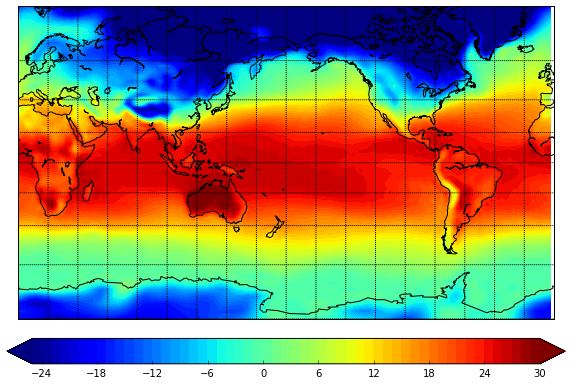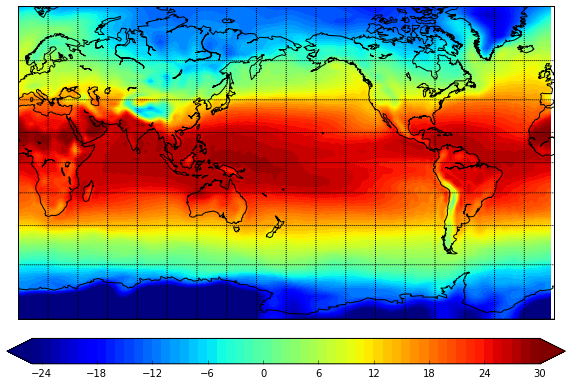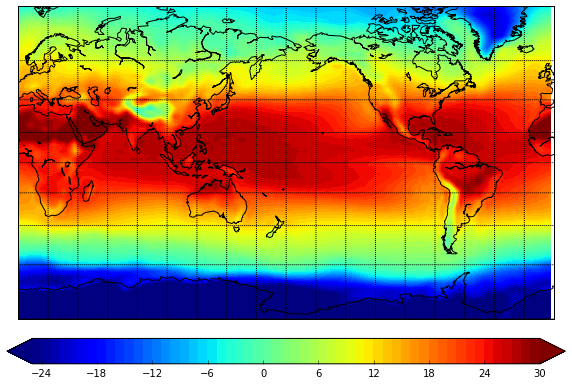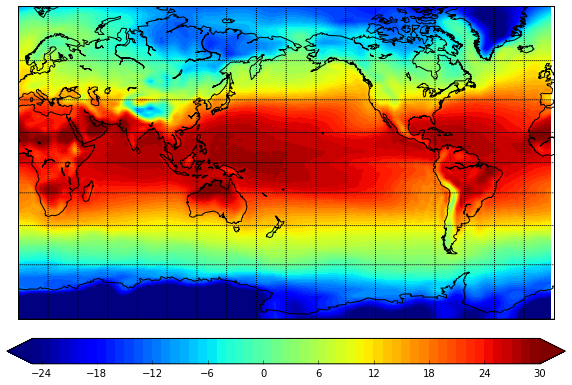# Select time ranges in multidimensional arrays with pandas

Select specific time ranges from multidimensional arrays



Solution

Pandas periods

I like pandas for very easy time handling, and would like to use similar approach when work with multidimensional arrays, for example from netCDF files. There are already some efforts to do this. However I don't need anything complicated, just select some months, years of time periods. For this I can use pandas itself and benefit from its great time indexing. Below I will show a small example of how to do this.

Necessary imports (everything can be installed from Anaconda)

In :
import matplotlib.pylab as plt
from netCDF4 import Dataset, num2date
import pandas as pd
from mpl_toolkits.basemap import Basemap
import numpy as np
%matplotlib inline


In :
#!wget ftp://ftp.cdc.noaa.gov/Datasets/ncep.reanalysis.derived/surface/air.mon.mean.nc


Open the file:

In :
f = Dataset('./air.mon.mean.nc')


Load variables, and create 2D lat and lon

In :
air = f.variables['air'][:]
time = f.variables['time']
lon = f.variables['lon'][:]
lat = f.variables['lat'][:]

lon, lat = np.meshgrid(lon, lat)


Here we create dates variable, that contains datetime values, extracted by num2date function from netCDF4 package (don't confuse it with matplotlib version, which is not that great!)

In :
dates = num2date(time[:], time.units)

In :
dates[:3]

Out:
array([datetime.datetime(1948, 1, 1, 0, 0),
datetime.datetime(1948, 2, 1, 0, 0),
datetime.datetime(1948, 3, 1, 0, 0)], dtype=object)

Now we convert dates to pandas format:

In :
dates_pd = pd.to_datetime(dates)


And then convert timestemps to periods, since we dealing with monthly values:

In :
periods = dates_pd.to_period(freq='M')

In :
periods

Out:

[1948-01, ..., 2015-01]
Length: 805, Freq: M

This is a small helper function, that will plot our results:

In :
def plt_map(data):
m = Basemap(projection='mill',llcrnrlat=-80,urcrnrlat=80,\
llcrnrlon=0,urcrnrlon=360,lat_ts=20,resolution='c')
x, y = m(lon, lat)
plt.figure(figsize=(10,7))
m.drawcoastlines()
m.drawparallels(np.arange(-80.,81.,20.))
m.drawmeridians(np.arange(-180.,181.,20.))
m.drawmapboundary(fill_color='white')
m.contourf(x,y,data,levels=np.linspace(-25,30,56), extend="both");


Test on the first time step:

In :
plt_map(air[0,:,:])## Select specific year (yearly mean)¶

We create the mask from the periods where only 2010 is selected, then apply it to the time dimension of our variable, and calculate the mean over time:

In :
mask_2014 = periods.year==2010

In :
data = air[mask_2014,:,:].mean(axis=0)

In :
plt_map(data)## Monthly mean¶

Here we do the same thing, but for months (September mean):

In :
mask_months = periods.month==9

In :
data = air[mask_months,:,:].mean(axis=0)

In :
plt_map(data)## Time period¶

Select only Octobers in 2000s:

In :
mask_tm = (periods.year>=2000)&(periods.year<=2010)&(periods.month==10)


And calculate the mean:

In :
data = air[mask_tm,:,:].mean(axis=0)

In :
plt_map(data)And so on... I guess you get the idea :)